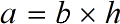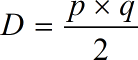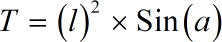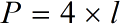Request a Tool

# Area of a rhombus Calculator

Select the method by which you want to calculate the area or parameter of the rhombus and then enter the required values

Area of Rhombus
0

#### Formula• a = Base Area of rhombus
• b = Area of rhombus
• h = Height of rhombus

#### Defination / Uses

A rhombus is a 2D shape have every side of equal length and equal opposite angle. A parallelogram with equal sides is also known as a rhombus. It's similar to a lean square with diagonals that bisect each other but aren't always 90 degrees. The shape of a rhombus is sometimes known as a 'diamond' or 'lozenge.' Rhombus has congruent sides on all four sides. It has perpendicular diagonals that intersect the vertex angles.

Area of Rhombus
0

#### Formula• D = Digonal Area of rhombus
• p = Digonal Length (p) of rhombus
• q = Digonal Length (q) of rhombus

#### Defination / Uses

A rhombus is a 2D shape have every side of equal length and equal opposite angle. A parallelogram with equal sides is also known as a rhombus. It's similar to a lean square with diagonals that bisect each other but aren't always 90 degrees. The shape of a rhombus is sometimes known as a 'diamond' or 'lozenge.' Rhombus has congruent sides on all four sides. It has perpendicular diagonals that intersect the vertex angles.

Area of Rhombus
0

#### Formula• T = Trignomatric Area of rhombus
• l = Length of rhombus
• a = Angle(a) of rhombus

#### Defination / Uses

A rhombus is a 2D shape have every side of equal length and equal opposite angle. A parallelogram with equal sides is also known as a rhombus. It's similar to a lean square with diagonals that bisect each other but aren't always 90 degrees. The shape of a rhombus is sometimes known as a 'diamond' or 'lozenge.' Rhombus has congruent sides on all four sides. It has perpendicular diagonals that intersect the vertex angles.

#### Output

Perameter of Rhombus
0

#### Formula• p = Perameter of rhombus
• l = Length of rhombus

#### Defination / Uses

A rhombus is a 2D shape have every side of equal length and equal opposite angle. A parallelogram with equal sides is also known as a rhombus. It's similar to a lean square with diagonals that bisect each other but aren't always 90 degrees. The shape of a rhombus is sometimes known as a 'diamond' or 'lozenge.' Rhombus has congruent sides on all four sides. It has perpendicular diagonals that intersect the vertex angles.

### How to calculte area of rhombus?

There are only two basic steps.

• First select the option from dropdown. What you need to calculate.
• Then add the other values in their corresponding fields.